### Asir-contrib-HG package to solve GKZ hypergeometric systems

GKZ hypergeometric system is a system of linear partial differential equations associated to(an integer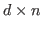-matrix of rank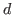) and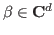. The book by Saito, Sturmfels and Takayama  discusses algorithmic methods to construct series solutions of the GKZ system. The current Asir-contrib-HG package is built in order to implement these algorithms. What we need for the implementation are mainly (1) Gröbner basis computation both in the ring of polynomials and in the ring of differential operators, and (2) enumeration of all the Gröbner bases of toric ideals. Asir and kan/sm1 provide functions for (1) and TiGERS provides a function for (2). These components communicate each other by OpenXM-RFC 100 protocol.

Let us see an example how to construct series solution of a GKZ hypergeometric system. The function dsolv_starting_term finds the leading terms of series solutions to a given direction.

1. Generate the GKZ hypergeometric system associated toby the function sm1_gkz.
 F = sm1_gkz(
[ [[1,1,1,1,1],
[1,1,0,-1,0],
[0,1,1,-1,0]], [1,0,0]]);
[[x5*dx5+x4*dx4+x3*dx3+x2*dx2+x1*dx1-1,
-x4*dx4+x2*dx2+x1*dx1,
-x4*dx4+x3*dx3+x2*dx2,
-dx2*dx5+dx1*dx3,dx5^2-dx2*dx4],
[x1,x2,x3,x4,x5]]

2. Find the leading terms of this system to the direction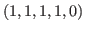.
 A= dsolv_starting_term(F,F,
[1,1,1,1,0])\$
Computing the initial ideal.
Done.
Computing a primary ideal decomposition.
Primary ideal decomposition of
the initial Frobenius ideal
to the direction [1,1,1,1,0] is
[[[x5+2*x4+x3-1,x5+3*x4-x2-1,
x5+2*x4+x1-1,3*x5^2+(8*x4-6)*x5-8*x4+3,
x5^2-2*x5-8*x4^2+1,x5^3-3*x5^2+3*x5-1],
[x5-1,x4,x3,x2,x1]]]

----------- root is [ 0 0 0 0 1 ]
----------- dual system is
[x5^2+(-3/4*x4-1/2*x3-1/4*x2-1/2*x1)*x5+1/8*x4^2
+(1/4*x3+1/4*x1)*x4+1/4*x2*x3-1/8*x2^2+1/4*x1*x2,
x4-2*x3+3*x2-2*x1,x5-x3+x2-x1,1]

3. From the output, we can see that we have four possible leading terms. Factoring these leading terms, we get the following simpler expressions. The third entry [[1,1],[x5,1],[-log(x1)+log(x2)-log(x3)+log(x5),1]], means that there exists a series solution which starts with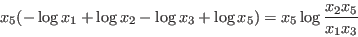A;
[[ 0 0 0 0 1 ]]
 map(fctr,A);
[[[1/8,1],[x5,1],[log(x2)+log(x4)-2*log(x5),1],
[2*log(x1)-log(x2)+2*log(x3)+log(x4)-4*log(x5)
,1]],
[[1,1],[x5,1],
[-2*log(x1)+3*log(x2)-2*log(x3)+log(x4),1]],
[[1,1],[x5,1],
[-log(x1)+log(x2)-log(x3)+log(x5),1]],
[[1,1],[x5,1]]]


Nobuki Takayama 2017-03-30